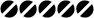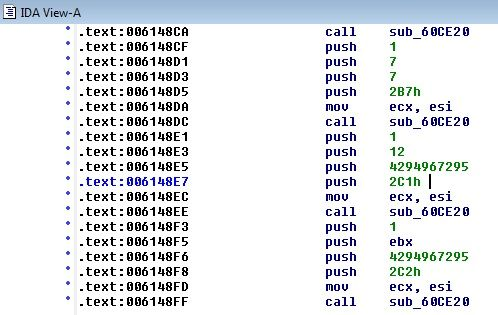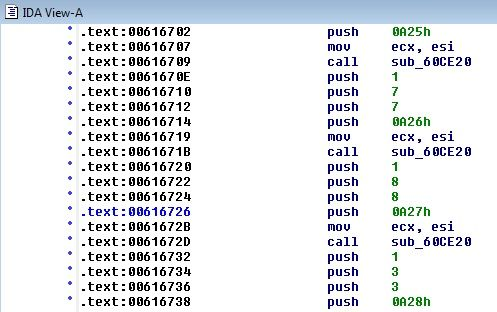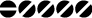# Openkore.com

OpenKore Forums
 It is currently 19 Feb 2020, 16:25

 All times are UTC - 5 hours [ DST ]Page 5 of 9 [ 90 posts ] Go to page Previous  1, 2, 3, 4, 5, 6, 7, 8, 9  Next
 Print view Previous topic | Next topic
Author Message
 Post subject: Re: Usage: Packet Extraction Evaluation Kit (PEEK)Posted: 11 Jan 2015, 18:46NoobJoined: 20 Mar 2011, 08:15
Posts: 18
@4epT

I' used the Packet Extractor-1.1.0 and RO Tools to extract recvpackets and the PEEK but is not working.

Can you give me that big help extracting the rcvpackets?
I found the server info and im logging in but i cant move or do anything.

The server is http://remember-ro.net/site/

Thanks alotTopPost subject: Re: Usage: Packet Extraction Evaluation Kit (PEEK)Posted: 12 Jan 2015, 07:21DevelopersJoined: 30 Apr 2008, 14:17
Posts: 624
Location: Moskow (Russia)
jokinvipo, from what file you are trying to get the package?

_________________
All my posts are made by machine translator!
¤ Manual ¤ Anti BotKiller ¤ Packet Extractor v3 ¤TopPost subject: Re: Usage: Packet Extraction Evaluation Kit (PEEK)Posted: 13 Jan 2015, 19:45NoobJoined: 20 Mar 2011, 08:15
Posts: 18
I tried to extract the launchers but is not working.

i only need the rcvpackets for this kRO_Sakexe_2008_09_10a then my bot should run w/o problems.

Can you give me a hand?

Thanks

TopPost subject: Re: Usage: Packet Extraction Evaluation Kit (PEEK)Posted: 17 Jan 2015, 16:44DevelopersJoined: 30 Apr 2008, 14:17
Posts: 624
Location: Moskow (Russia)
jokinvipo, use "remember-ro.bin" and "openkore-packet-extractor-1.1.0"

_________________
All my posts are made by machine translator!
¤ Manual ¤ Anti BotKiller ¤ Packet Extractor v3 ¤TopPost subject: Re: Usage: Packet Extraction Evaluation Kit (PEEK)Posted: 17 Jan 2015, 22:18NoobJoined: 17 Jan 2015, 20:45
Posts: 8
sir, i got Unknown switch
# Packet Parser: Unknown switch: 02C1
# Packet Tokenizer: Unknown switch: 0A27

a tried already with tools from http://wiki.openkore.com/index.php/Packet_Length_Extractor, but failed (over 6MB)
also tried with PEEK, could you help...

then i tried with IDA, and search text manually02C1 4294967295 12 1
0A27 8 8 1

but the value seem to high
https://www.mediafire.com/?p11m940g4261z1g

TopPost subject: Re: Usage: Packet Extraction Evaluation Kit (PEEK)Posted: 18 Jan 2015, 06:18NoobJoined: 06 Feb 2009, 07:28
Posts: 17
Hello guys, I'm trying to use this application. But it seems I'm kinda confused with the exes that which I am going to use. I've read times over times but I'm not getting this. So I'll write down what I have and I would like to know what I have to do. (I'm trying to log on to fRo servers btw via OpenKore)

I've extracted PEEK into the RO folder itself with all the files that are included within PEEKHere are the original game files that I am choosing for PEEK to work on.After this point when I hit "Start Analyzer" button, I get the error message;I'm sure that I'm doing something wrong, but I cannot figure it out yet. If you guys can help me kindly, I'll be glad ! (Also will post the fRO's updated recvtables if I succeed. Or more than that I can also share the files if needed.)

TopPost subject: Re: Usage: Packet Extraction Evaluation Kit (PEEK)Posted: 19 Jan 2015, 08:59HumanJoined: 22 Feb 2013, 10:18
Posts: 22
i've tried to extract this but got this packet error.here's the exe.
http://www.4shared.com/file/9xnj0iZ9ce/LeafRO.html

TopPost subject: Re: Usage: Packet Extraction Evaluation Kit (PEEK)Posted: 20 Jan 2015, 05:14DevelopersJoined: 30 Apr 2008, 14:17
Posts: 624
Location: Moskow (Russia)
Shakti-lar, "Unknown switch" - This means that you are using is not the right serveType. Check your file recvpackets.txt
you have to find "02C1 0" and "0A27 8". By the way how you extracted the packages?

tmrcik, Try to use other extractors.

_________________
All my posts are made by machine translator!
¤ Manual ¤ Anti BotKiller ¤ Packet Extractor v3 ¤TopPost subject: Re: Usage: Packet Extraction Evaluation Kit (PEEK)Posted: 26 Jan 2015, 19:09NoobJoined: 26 Jan 2015, 12:58
Posts: 6
Hello people of this community =D

4epT wrote:
https://github.com/ThemonChan/PEEK
http://nbsxey3vnrsxgltxom.cmle.ru/board ... -analyzer/

Mini guide:
2. run PEEK.exe
3. click "Browse" and select your client file (not necessarily exe!)
4. click "Start Analyzer"
5. close PEEK
6. open folder: ..\PEEK\Output\
7. run 1.bat
8. file will appear: recvpackets.txt

i followed this guide to get the recvpackets.txt but when i get to step 7, i cant find any bat file in the entire PEEK folder.
Maybe its something you have to download from the second link, but i cant access that site. It makes me go to this site: http://cameleo.ru/?msg=edsina wich is in some language i cant understand and the google translations doesnt make any sense... =\
Including the bat file that is required in step 7?

Anyway i still get a recvpackets_20120515.txt file wich contains the following:

Code:
//Extracted With DiffGen2

[Old_Table]
0x0187 = 6
0x0081 = 3
0x01c6 = 4
0x01c7 = 2
0x0064 = 55
0x0069 = -1
0x006a = 23
0x01db = 2
0x01dc = -1
0x01dd = 47
0x01fa = 48
0x0204 = 18
0x01f1 = -1
0x0200 = 26
0x01be = 2
0x01bf = 3
0x0065 = 17
0x0066 = 3
0x0067 = 37
0x0970 = 31
0x020d = -1
0x006b = -1
0x006c = 3
0x006d = 146
0x006e = 3
0x0071 = 28
0x0068 = 46
0x01fb = 56
0x006f = 2
0x0070 = 3
0x02ca = 3
0x009b = 34
0x0082 = 2
0x00a7 = 9
0x0190 = 23
0x00f3 = -1
0x0089 = 11
0x007d = 2
0x0090 = 7
0x0099 = -1
0x019c = -1
0x0085 = 10
0x00cc = 6
0x00ce = 2
0x00f5 = 11
0x0116 = 17
0x009f = 20
0x00a9 = 6
0x00ab = 4
0x00b8 = 7
0x00b9 = 6
0x00b2 = 3
0x00ba = 2
0x00bb = 5
0x00bf = 3
0x00c1 = 2
0x00c5 = 7
0x00c8 = -1
0x00c9 = -1
0x0096 = -1
0x00cf = 27
0x00d0 = 3
0x00d3 = 2
0x00d5 = -1
0x00d9 = 14
0x00de = -1
0x00e0 = 30
0x00e2 = 26
0x00e3 = 2
0x00e4 = 6
0x00e6 = 3
0x00e8 = 8
0x00eb = 2
0x00ed = 2
0x00ef = 2
0x0094 = 19
0x00f7 = 17
0x0193 = 2
0x00f9 = 26
0x01e8 = 28
0x00fc = 6
0x00ff = 10
0x0102 = 6
0x0100 = 2
0x0103 = 30
0x0108 = -1
0x0112 = 4
0x0072 = 22
0x0113 = 25
0x0118 = 2
0x011b = 20
0x011d = 2
0x0126 = 8
0x0127 = 8
0x0128 = 8
0x0129 = 8
0x012a = 2
0x012e = 2
0x012f = -1
0x01b2 = -1
0x0134 = -1
0x0130 = 6
0x0138 = 3
0x013f = 26
0x0140 = 22
0x0143 = 10
0x0146 = 6
0x0178 = 4
0x017a = 4
0x017c = 6
0x01fd = 15
0x018a = 4
0x018e = 10
0x007e = 105
0x0197 = 4
0x0198 = 8
0x01ae = 4
0x025b = 6
0x01af = 4
0x01b9 = 6
0x01cd = 30
0x01ce = 6
0x0443 = 8
0x01cf = 28
0x01d0 = 8
0x01e1 = 8
0x01d1 = 14
0x01d2 = 10
0x008c = 14
0x0095 = 30
0x0195 = 102
0x00a2 = 14
0x0175 = 6
0x0176 = 106
0x0079 = 53
0x019d = 6
0x014c = -1
0x014d = 2
0x014e = 6
0x014f = 6
0x0150 = 110
0x01b6 = 114
0x0151 = 6
0x0152 = -1
0x0153 = -1
0x0154 = -1
0x0166 = -1
0x0155 = -1
0x0156 = -1
0x0157 = 6
0x0159 = 54
0x015a = 66
0x015b = 54
0x015c = 90
0x015d = 42
0x015e = 6
0x015f = 42
0x0160 = -1
0x0161 = -1
0x0162 = -1
0x0163 = -1
0x0164 = -1
0x0165 = 30
0x0167 = 3
0x0168 = 14
0x0169 = 3
0x016a = 30
0x016b = 10
0x0149 = 9
0x014a = 6
0x014b = 27
0x016e = 186
0x016f = 182
0x017e = -1
0x017f = -1
0x0165 = 30
0x0166 = -1
0x0167 = 3
0x0168 = 14
0x0169 = 3
0x016a = 30
0x016b = 10
0x016c = 43
0x016c = 43
0x016d = 14
0x01f2 = 20
0x0170 = 14
0x0171 = 30
0x0172 = 10
0x0173 = 3
0x0174 = -1
0x0180 = 6
0x0181 = 3
0x0182 = 106
0x0183 = 10
0x0184 = 10
0x0185 = 34
0x019e = 2
0x019f = 6
0x01a0 = 3
0x01a1 = 3
0x01a2 = 37
0x01a3 = 5
0x01a4 = 11
0x01a5 = 26
0x01a6 = -1
0x01a7 = 4
0x01a8 = 4
0x01a9 = 6
0x01aa = 10
0x01ca = 3
0x01b0 = 11
0x01b1 = 7
0x01ba = 26
0x01bb = 26
0x01bc = 26
0x01bd = 26
0x01c0 = 2
0x01c1 = 14
0x01c2 = 10
0x01d3 = 35
0x01d5 = -1
0x01d4 = 6
0x01df = 6
0x01f3 = 10
0x0284 = 14
0x01ff = 10
0x01ed = 2
0x01e7 = 2
0x01b7 = 6
0x01f7 = 14
0x01e3 = 14
0x01cb = 9
0x01f9 = 6
0x01e5 = 6
0x0201 = -1
0x0369 = 26
0x0203 = 10
0x0205 = 26
0x0206 = 11
0x0207 = 34
0x0208 = 14
0x0209 = 36
0x020a = 10
0x020e = 32
0x020f = 10
0x0210 = 22
0x0212 = 26
0x0213 = 26
0x0214 = 42
0x0215 = 6
0x0216 = 6
0x0217 = 2
0x0218 = 2
0x0225 = 2
0x0966 = 4
0x0219 = 282
0x021a = 282
0x0226 = 282
0x0282 = 284
0x021b = 10
0x021c = 10
0x0224 = 10
0x0280 = 12
0x0285 = 6
0x0286 = 4
0x021d = 6
0x021e = 6
0x021f = 66
0x0222 = 6
0x0221 = -1
0x0220 = 10
0x0223 = 8
0x0073 = 11
0x0074 = 3
0x0075 = -1
0x0076 = 9
0x0077 = 5
0x0078 = 55
0x007a = 58
0x007b = 60
0x007c = 44
0x007f = 6
0x0080 = 7
0x0083 = 2
0x0084 = 2
0x0086 = 16
0x0087 = 12
0x0088 = 10
0x08cd = 10
0x008a = 29
0x008b = 23
0x008d = -1
0x008e = -1
0x0091 = 22
0x0092 = 28
0x0093 = 2
0x0097 = -1
0x0098 = 3
0x009a = -1
0x009c = 9
0x009d = 17
0x009e = 17
0x00a0 = 23
0x00a1 = 6
0x00a3 = -1
0x00a4 = -1
0x00a5 = -1
0x00a6 = -1
0x00a8 = 7
0x00aa = 9
0x00ac = 7
0x00ae = -1
0x00af = 6
0x00b0 = 8
0x00b1 = 8
0x00b3 = 3
0x00b4 = -1
0x00b5 = 6
0x00b6 = 6
0x00b7 = -1
0x00bc = 6
0x00bd = 44
0x00be = 5
0x00c0 = 7
0x00c2 = 6
0x00c3 = 8
0x00c4 = 6
0x00c6 = -1
0x00c7 = -1
0x00ca = 3
0x00cb = 3
0x00cd = 3
0x00d1 = 4
0x00d2 = 4
0x00d4 = -1
0x00d6 = 3
0x00d7 = -1
0x00d8 = 6
0x00da = 3
0x00db = -1
0x00dc = 28
0x00dd = 29
0x00df = -1
0x00e1 = 30
0x00e5 = 26
0x00e7 = 3
0x00e9 = 19
0x00ea = 5
0x00ec = 3
0x00ee = 2
0x00f0 = 3
0x00f1 = 2
0x00f2 = 6
0x00f4 = 21
0x00f6 = 8
0x00f8 = 2
0x00fa = 3
0x00fb = -1
0x00fd = 27
0x00fe = 30

[Encryption]
Key1 = 0x4a715ef9
Key2 = 0x79103e4f
Key3 = 0x405c1238

I cutted most part of the Old Table from the code in order to make it easy to read the post.

This didnt work as a recvpackets.txt for my server so i looked up and in the 2nd post of this topic the Moderator Skylord said there was a need to place a filename.pl file with the code he shared on the output paste of PEEK. and then run "it" in order to make the convertion automatic.

Quote:
Save as filename.pl '-' Inside Output folder of PEEK. Now run it!

Should i place this .pl file in the Output paste before i run the PEEK?
If yes, should i create the paste Output to put it there or...?
If no, what is the "it" that i should run?

TopPost subject: Re: Usage: Packet Extractor v3Posted: 30 Jan 2015, 20:59NoobJoined: 30 Jan 2015, 20:54
Posts: 1
hi....
it wont work with my client
thanks

here my file

TopDisplay posts from previous: All posts1 day7 days2 weeks1 month3 months6 months1 year Sort by AuthorPost timeSubject AscendingDescendingPage 5 of 9 [ 90 posts ] Go to page Previous  1, 2, 3, 4, 5, 6, 7, 8, 9  Next

 All times are UTC - 5 hours [ DST ]

#### Who is online

Users browsing this forum: No registered users and 1 guest

 You cannot post new topics in this forumYou cannot reply to topics in this forumYou cannot edit your posts in this forumYou cannot delete your posts in this forumYou cannot post attachments in this forum

Search for:
 Jump to:  Select a forum ------------------ Announcements    Announcements Support    Frequently Asked Questions & Guides    Resolved Questions    Official Servers       bRO       fRO       iRO       idRO       kRO       jRO       tRO       twRO       pRO       Private Servers       Other OpenKore support Discussions    Discussion    Tips & Tricks    Feature suggestions Plugins    Howto write plugins    Macro Plugin       Share your macro's    Other Plugins Technical    Developers Corner    Development Help    Tester's corner    Bug reports       Fixed bugs       Unresolvable bugs    Commit Queue Off Topic    Misc    Trashcan Links    OpenKore Manual    Official GitHub    Download Openkore .zip    AJAX IRC Client (Chat)    Developer Manual